Chapter 2.1 (Demo)

# Circle. Circumference

• What is the diameter of the circle?
• How to calculate the circumference?

## Radius and diameter of the circle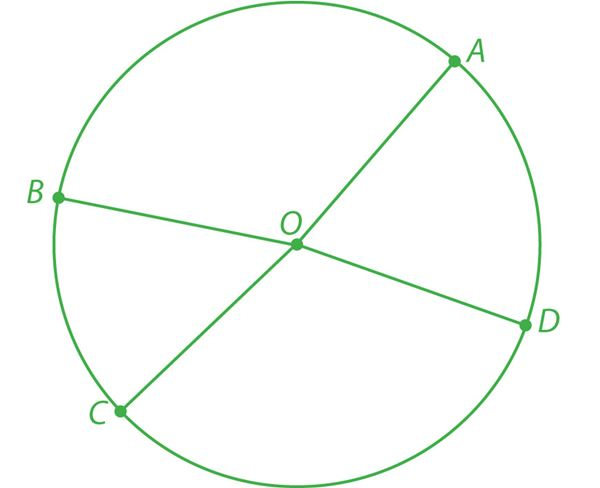OA =  mm

OB =  mm

OC =  mm

OD =  mm

The history of the compass

Since the section between the endpoints of the compass arms was the same all the time, the measurement also confirmed that all points of the circle are equidistant from the point O. This point is called the center of the circle.

All points of the circle are at the same distance from the center of the circle.

The section connecting the center of a circle to any point on the circle, and also the length of that section, is called the radius of the circle.

The radius is denoted by the letter r.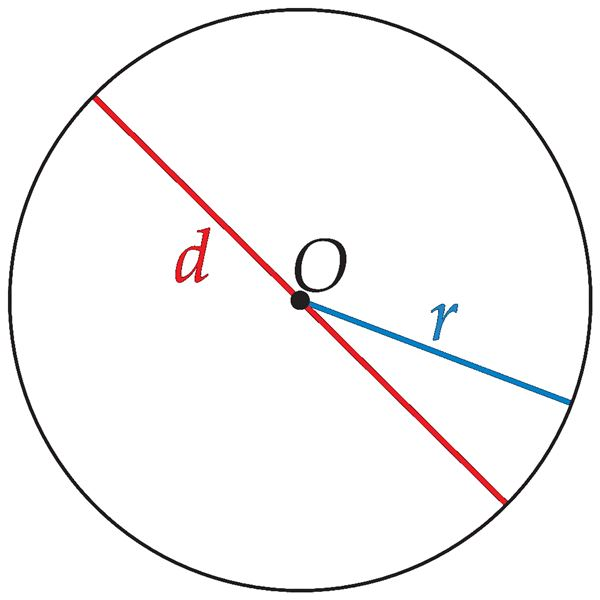The center, radius, and diameter of the circle

The section that passes through the center of the circle and connects the two points of the circle is called the diameter of the circle.

The diameter is denoted by the letter d and is twice as long as the radius, so d = 2r.

 Radius 2.5 cm 0.4 dm cm dm Diameter cm dm 10.8 cm 0.5 dm

## Circumference

In the distant past, scientists have noticed that any circle is a certain number of times longer than its diameter. This number is denoted by the Greek lowercase letter π (pronounce: pie).

The length of the circle is denoted by the letter C.

Here we get the formula for the length of the circle.

C = πd

The length of the circle is π times the diameter.

Since the diameter of the circle is twice as long as the radius, ie d = 2r, we can also write as follows:

C = 2πr

The ancient Greek scientist Archimedes (287–212 BC) determined that the value of π is between . The number π is an infinite non-periodic decimal fraction, the first few places of which are as follows: π = 3.14159265358979 …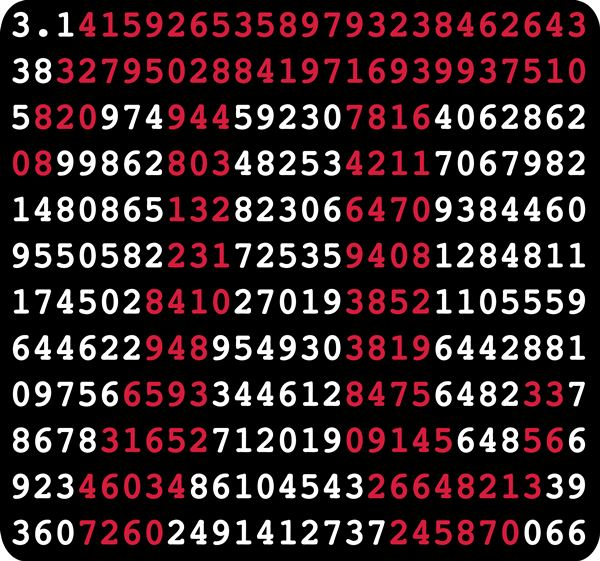#### Interesting to Know

March 14 is the international π‑day (the 14th day of third month).

The day was first celebrated in 1988 at the San Francisco Science Park, where attendees circled and ate apple pie. The reason is that in English, π and pie (cake) are pronounced similarly.

Those interested in mathematics will compete to remember π decimal places that day, the current record is over 67 000 decimal places. It belongs to the Chinese, Lu Chao. It took him 24 hours and four minutes to read Pi's 67 890 decimal places.

Use the approximate value of π to solve the problems.

π ≈ 3.14 or π ≈ $\frac{22}{7}$

#### Example

Let's calculate the length of the circle if the diameter is 7 cm.

C = πd

C ≈ 3.14 · 7 ≈ 21.98 (cm)

or

C ≈ $\frac{22}{7}$ · 7 ≈ 22 (cm)

## I'm experimenting and researching

 CircumferenceC Diameterd $\frac{C}{d}$

Compare the resulting quotients with the value of π. What do you notice?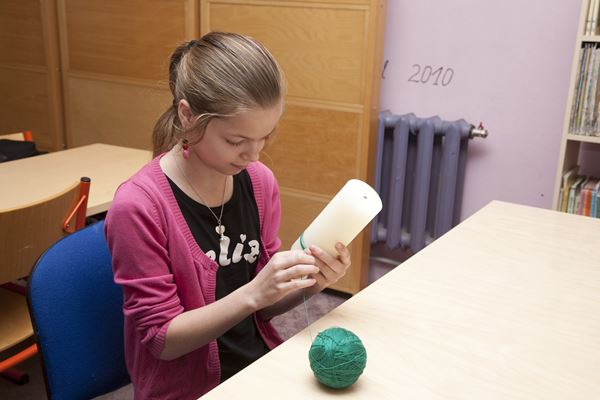Recommendation. For a more accurate circumference, wind several threads of wire tightly next to each other and divide the resulting length by the number of turns.

## *Mathematics club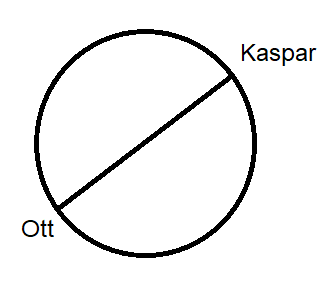Kaspar has completed  laps by then.

## Formulas

C = πd

C = 2πr

π = 3.14

d = 2r

r = d : 2

C – circumference

d – diameter